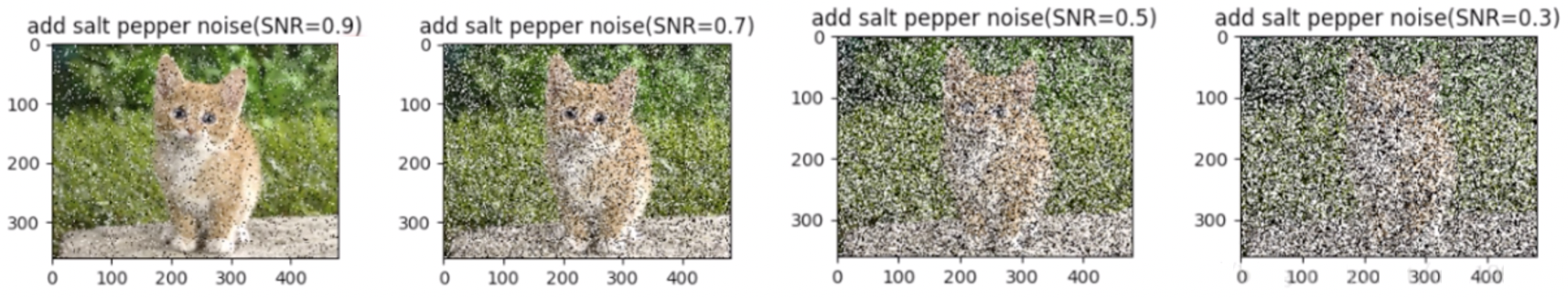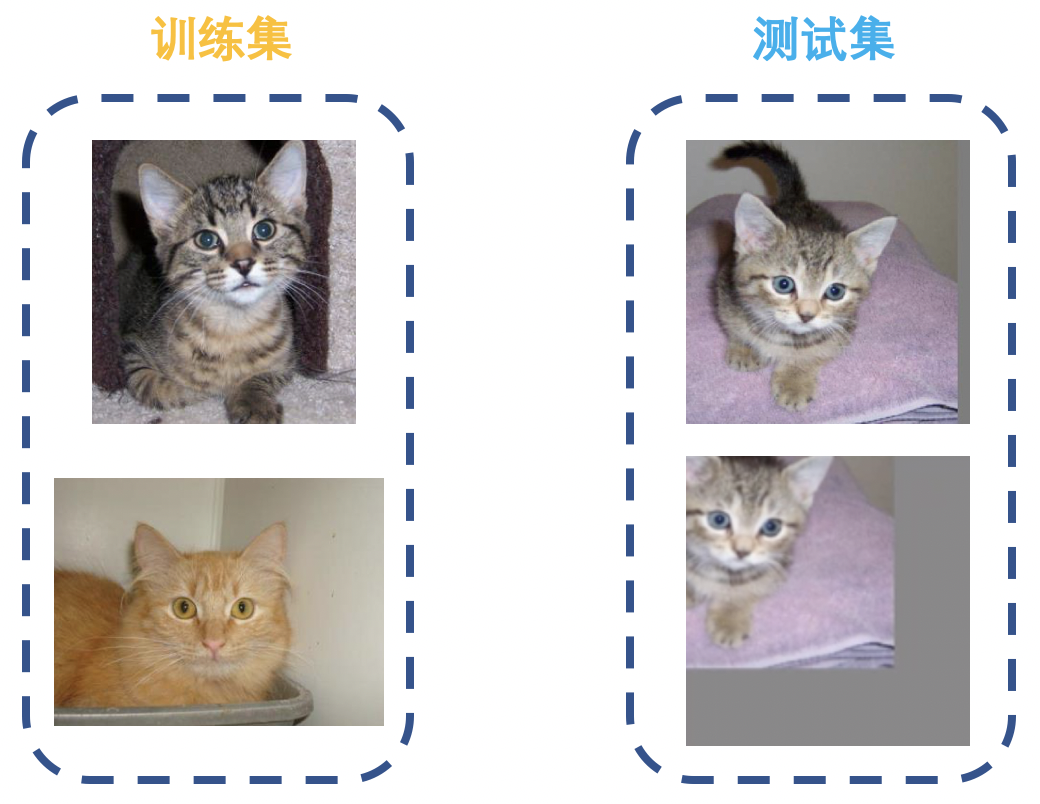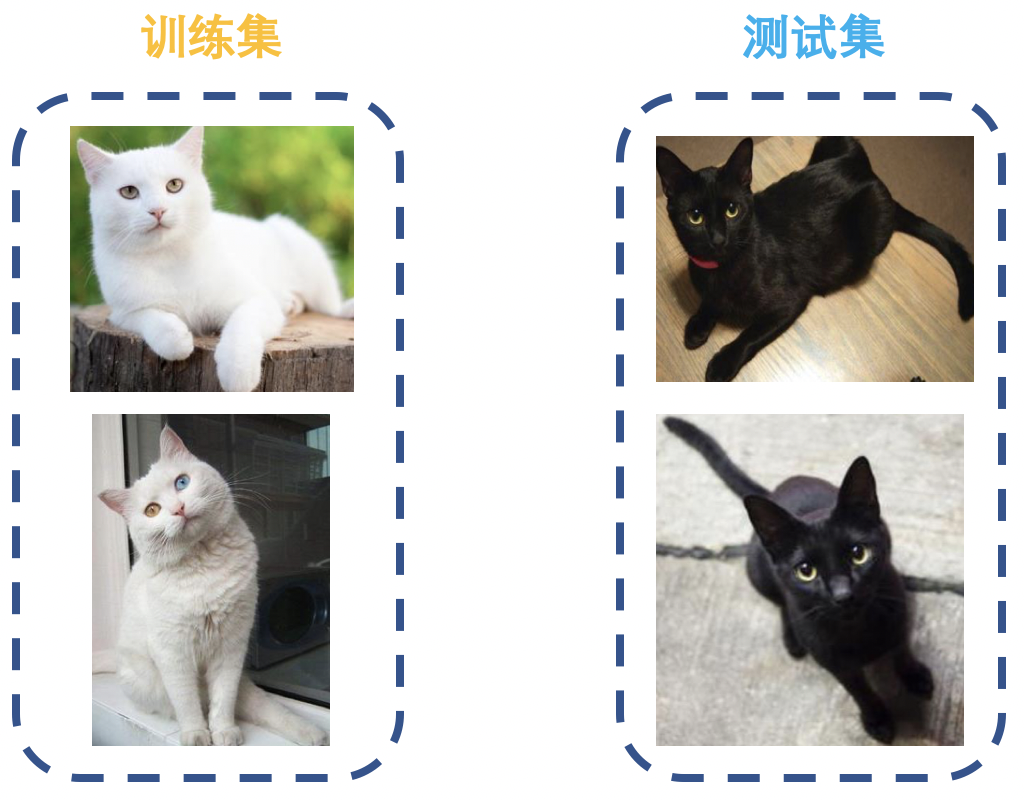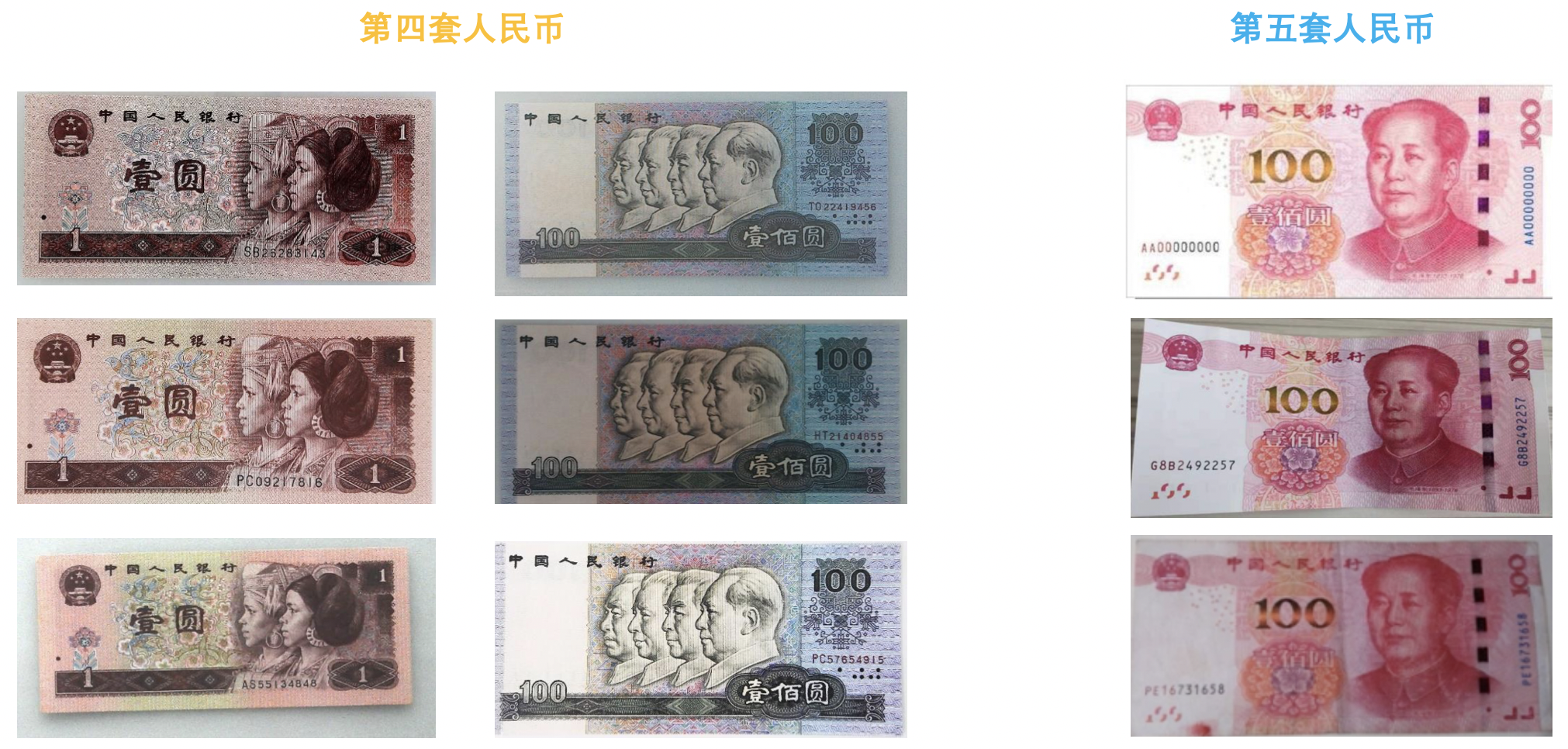# PyTorch 09：transforms 图像变换、方法操作及自定义方法

## PyTorch 学习笔记

Posted by YEY on December 13, 2020

# Lecture 09 transforms 图像变换、方法操作及自定义方法

## 1. transforms 图像变换

#### transforms.Pad

1
2
3
4
5
fill=0,
)


• padding：设置填充大小。
• 当为 a 时，上下左右均填充 a 个像素。
• 当为 (a, b) 时，上下填充 b 个像素，左右填充 a 个像素。
• 当为 (a, b, c, d) 时，左、上、右、下分别填充 abcd 个像素。
• padding_mode：填充模式，有 4 种模式：
• constant
• edge
• reflect
• symmetric
• fill：当 padding_mode='constant' 时，设置填充的像素值，(R, G, B)(Gray)

#### transforms.ColorJitter

1
2
3
4
5
6
transforms.ColorJitter(
brightness=0,
contrast=0,
saturation=0,
hue=0
)


• brightness：亮度调整因子。
• 当为 a 时， 从 [max(0, 1-a), 1+a] 中随机选择。
• 当为 (a, b) 时， 从 [a, b] 中随机选择。
• contrast ：对比度参数，同 brightness
• saturation：饱和度参数，同 brightness
• hue：色相参数。
• 当为 a 时，从 [-a, a] 中选择参数。注：0 <= a <= 0.5
• 当为 (a, b) 时，从 [a, b] 中选择参数。注：-0.5 <= a <= b <= 0.5

#### transforms.Grayscale

1
transforms.Grayscale(num_output_channels)


• num_ouput_channels：输出通道数，只能设为 1 或 3。

#### transforms.RandomGrayscale

1
2
3
4
transforms.RandomGrayscale(
num_output_channels,
p=0.1
)


• num_ouput_channels：输出通道数，只能设为 1 或 3。
• p：概率值，图像被转换为灰度图的概率。

#### transforms.RandomAffine

1
2
3
4
5
6
7
8
transforms.RandomAffine(
degrees,
translate=None,
scale=None,
shear=None,
resample=False,
fillcolor=0
)


• degrees：旋转角度设置。
• translate：平移区间设置，如 (a, b), a 设置宽 (width)，b 设置高 (height)。图像在宽维度平移的区间为 -img_width * a < dx < img_width * a
• scale：缩放比例 (以面积为单位)。
• fill_color：填充颜色设置。
• shear：错切角度设置，有水平错切和垂直错切。
• 若为 a，则仅在 x 轴错切，错切角度在 (-a, a) 之间。
• 若为 (a, b)，则 a 设置 x 轴角度，b 设置 y 轴角度。
• 若为 (a, b, c, d)，则 ab 设置 x 轴角度，cd 设置 y 轴角度。
• resample：重采样方式，有 NEARESTBILINEARBICUBIC 三种。

#### transforms.RandomErasing

1
2
3
4
5
6
7
transforms.RandomErasing(
p=0.5,
scale=(0.02, 0.33),
ratio=(0.3, 3,3),
value=0,
inplace=False
)


• p：概率值，执行该操作的概率。
• scale：遮挡区域的面积。
• ratio：遮挡区域长宽比。
• value：设置遮挡区域的像素值，(R, G, B) 或者 (Gray)#### transforms.Lambda

1
transforms.Lambda(lambd)


• lambd：lambda 匿名函数，lambda [arg1 [,arg2, ... , argn]]: expression

1
2
transforms.TenCrop(200, vertical_flip=True),
transforms.Lambda(lambda crops: torch.stack([transforms.Totensor()(crop) for crop in crops]))


## 2. transforms 选择操作

#### transforms.RandomChoice

1
transforms.RandomChoice([transforms1, transforms2, transforms3])


#### transforms.RandomApply

1
transforms.RandomApply([transforms1, transforms2, transforms3], p=0.5)


#### transforms.RandomOrder

1
transforms.RandomOrder([transforms1, transforms2, transforms3])


## 3. 自定义 transforms

1
2
3
4
5
class Compose(object):
def __call__(self, img):
for t in self.transforms:
img = t(img)
return img


1. 仅接收一个参数，返回一个参数。
2. 注意上下游的输出与输入之间的数据类型必须要匹配。

1
2
3
4
5
6
class YourTransforms(object):
def __init__(self, ...):
...
def __call__(self, img):
...
return img


#### 例子：椒盐噪声1
2
3
4
5
6
7
8
9
10
11

def __init__(self, snr, p):
self.snr = snr  # 设置信噪比
self.p = p  # 设置概率值

def __call__(self, img):

...  # 添加椒盐噪声具体实现过程

return img


Python 代码示例

1
2
3
4
5
6
7
8
9
10
11
12
13
14
15
16
17
18
19
20
21
22
23
24
25
26
27
28
29
30
31
"""增加椒盐噪声
Args:
snr （float）: 信噪比，Signal Noise Rate
p (float): 概率值，依概率执行该操作
"""

def __init__(self, snr, p=0.9):
assert isinstance(snr, float) or (isinstance(p, float))
self.snr = snr
self.p = p

def __call__(self, img):
"""
Args:
img (PIL Image): PIL Image
Returns:
PIL Image: PIL image.
"""
if random.uniform(0, 1) < self.p:
img_ = np.array(img).copy()
h, w, c = img_.shape
signal_pct = self.snr
noise_pct = (1 - self.snr)
mask = np.random.choice((0, 1, 2), size=(h, w, 1), p=[signal_pct, noise_pct/2., noise_pct/2.])
img_[mask == 1] = 255   # 盐噪声
img_[mask == 2] = 0     # 椒噪声
return Image.fromarray(img_.astype('uint8')).convert('RGB')
else:
return img


## 4. transforms 方法总结

• transforms.CenterCrop
• transforms.RandomCrop
• transforms.RandomResizedCrop
• transforms.FiveCrop
• transforms.TenCrop

• transforms.RandomHorizontalFlip
• transforms.RandomVerticalFlip
• transforms.RandomRotation

• transforms.Pad
• transforms.ColorJitter
• transforms.Grayscale
• transforms.RandomGrayscale
• transforms.RandomAffine
• transforms.LinearTransformation
• transforms.RandomErasing
• transforms.Lambda
• transforms.Resize
• transforms.Totensor
• transforms.Normalize

transforms 操作

• transforms.RandomChoice
• transforms.RandomApply
• transforms.RandomOrder

## 5. 数据增强实战应用

• 空间位置：平移
• 色彩：灰度图，色彩抖动
• 形状：仿射变换
• 上下文场景：遮挡， 填充
• ……#### 人民币分类## 6. 总结本作品采用知识共享署名-非商业性使用-相同方式共享 4.0 国际许可协议进行许可。 欢迎转载，并请注明来自：YEY 的博客 同时保持文章内容的完整和以上声明信息！